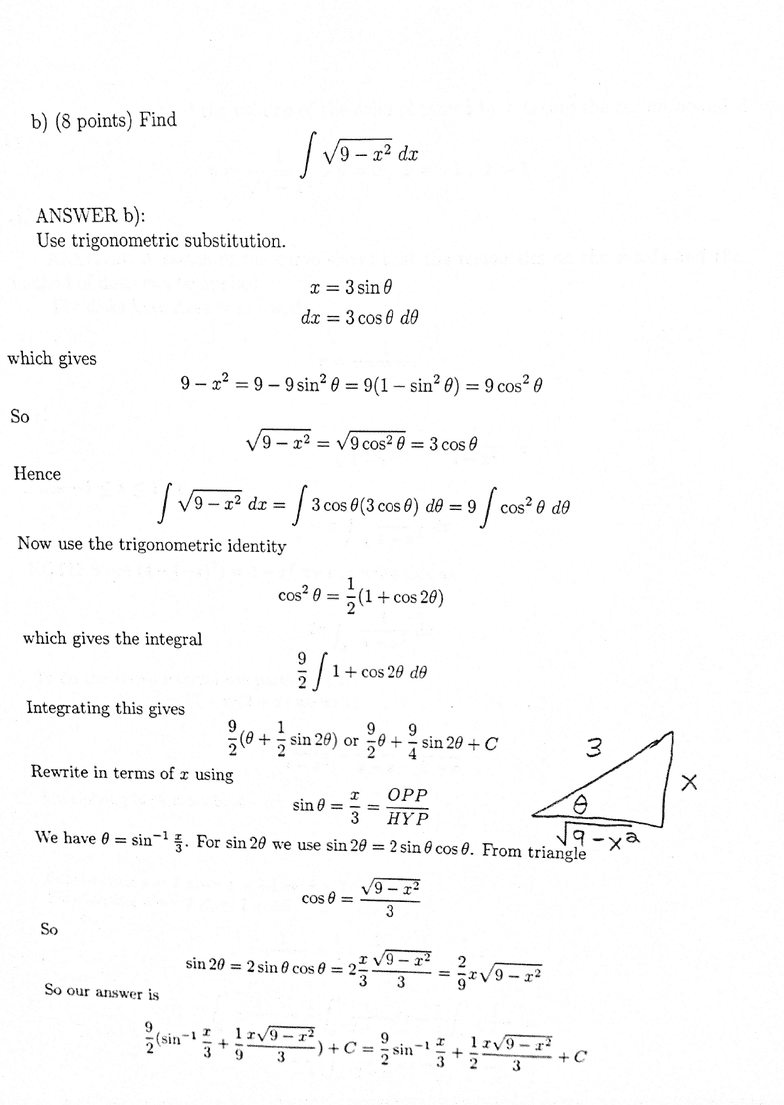# CAS MA 124 Study Guide - Midterm Guide: Ion, Horse Length, Partial Fraction Decomposition

156 views11 pages
School
ProfessorMA 124 - Fall ’12 - Exam 1A - Answers
1) (16 points) When given as a medication, iodine has a half-life in the bloodstream
of 8 days.
a) A patient is given an initial dose of 80 millicuries. Find the function that gives the
amount in the body after tdays.
b) How long does it take for the amount of iodine to reach 20% of the original dose ?
A(t)=A0ekt = 80ekt
Need k
Using the formula
k=ln 2
t1/2
=ln 2
8
which can be obtained by
A(8) = 40 = 80ek81
2=ek8
ln 1
2=lnek8=k8k=ln 1
2
8=ln 2
8
So
A(t) = 80e
ln 2
8t
b) Solve
.2(80) = 16 = 80e
ln 2
8t
I.e.
.2=e
ln 2
8t
ln(.2) = ln e
ln 2
8t=ln 2
8t
So
t=8ln(.2)
ln 2 =8 ln(1/5)
ln 2 =8ln5
ln 2
Unlock document

This preview shows pages 1-3 of the document.
Unlock all 11 pages and 3 million more documents.2) a) (8 points) Find
e
1
xln xdx
ANSWER a) Use integration by parts with
u=lnxdv=xdx
du =1
xdx v =xdx=1
2x2
udv=uv vdu
gives
xln xdx=1
2x2ln x1
2xdx
=1
2x2ln x1
2·1
2x2
=1
2x2ln x1
4x2
So e
1
xln xdx=1
2e2ln e1
4e2(1
2ln 1 1
4)
=1
2e21
4e2(1
4)
=1
4e2+1
4
=1
4(e2+ 1)
Unlock document

This preview shows pages 1-3 of the document.
Unlock all 11 pages and 3 million more documents.Unlock document

This preview shows pages 1-3 of the document.
Unlock all 11 pages and 3 million more documents.